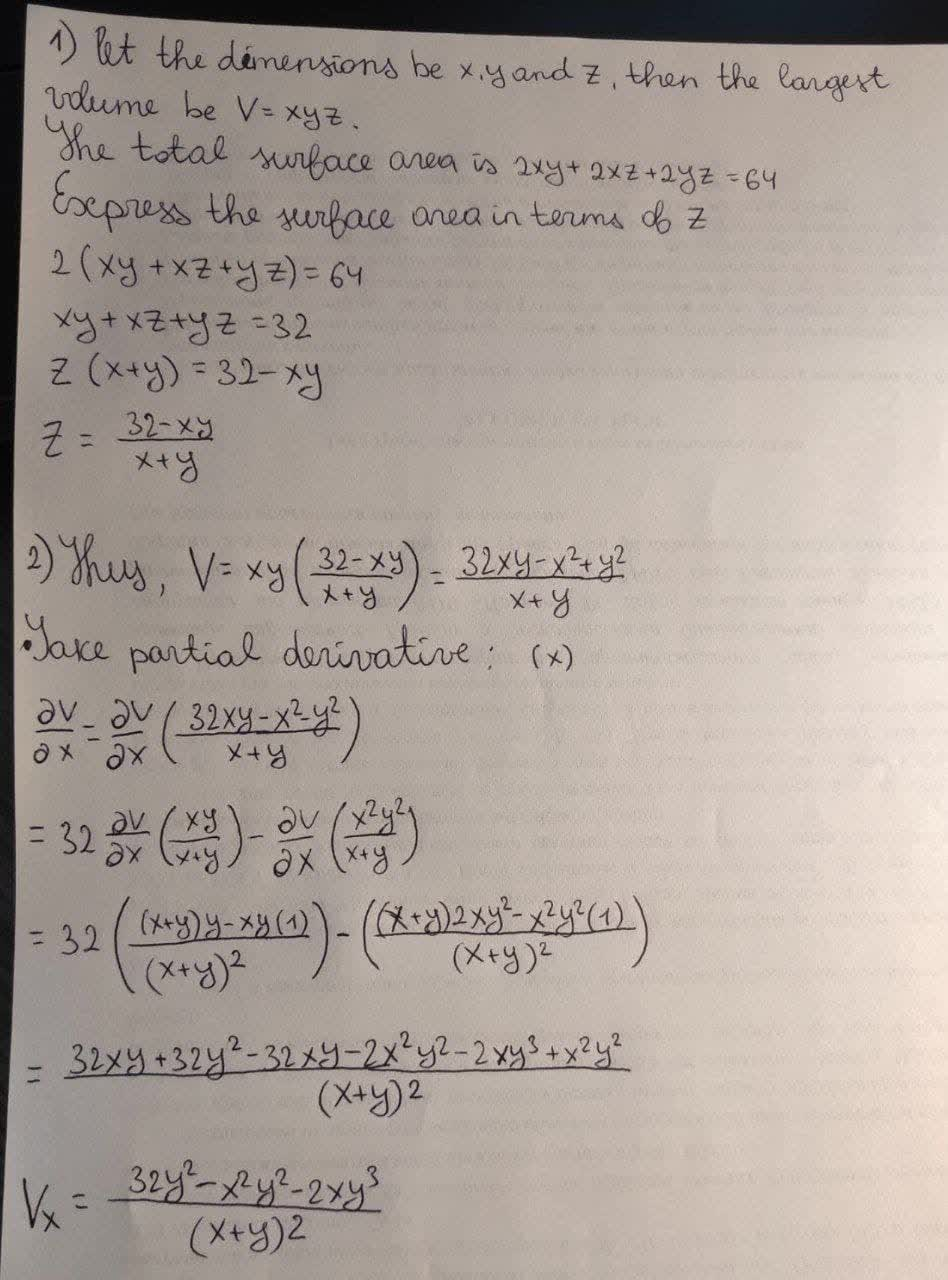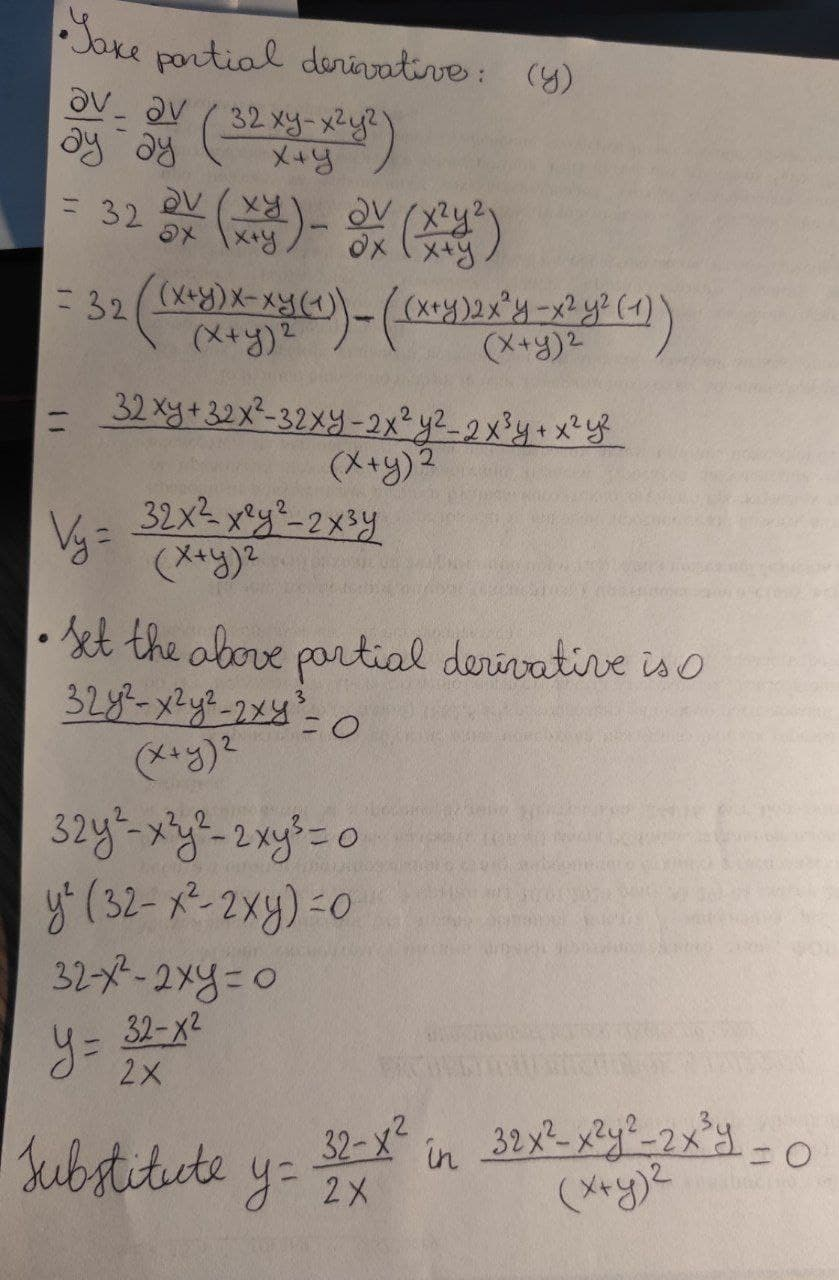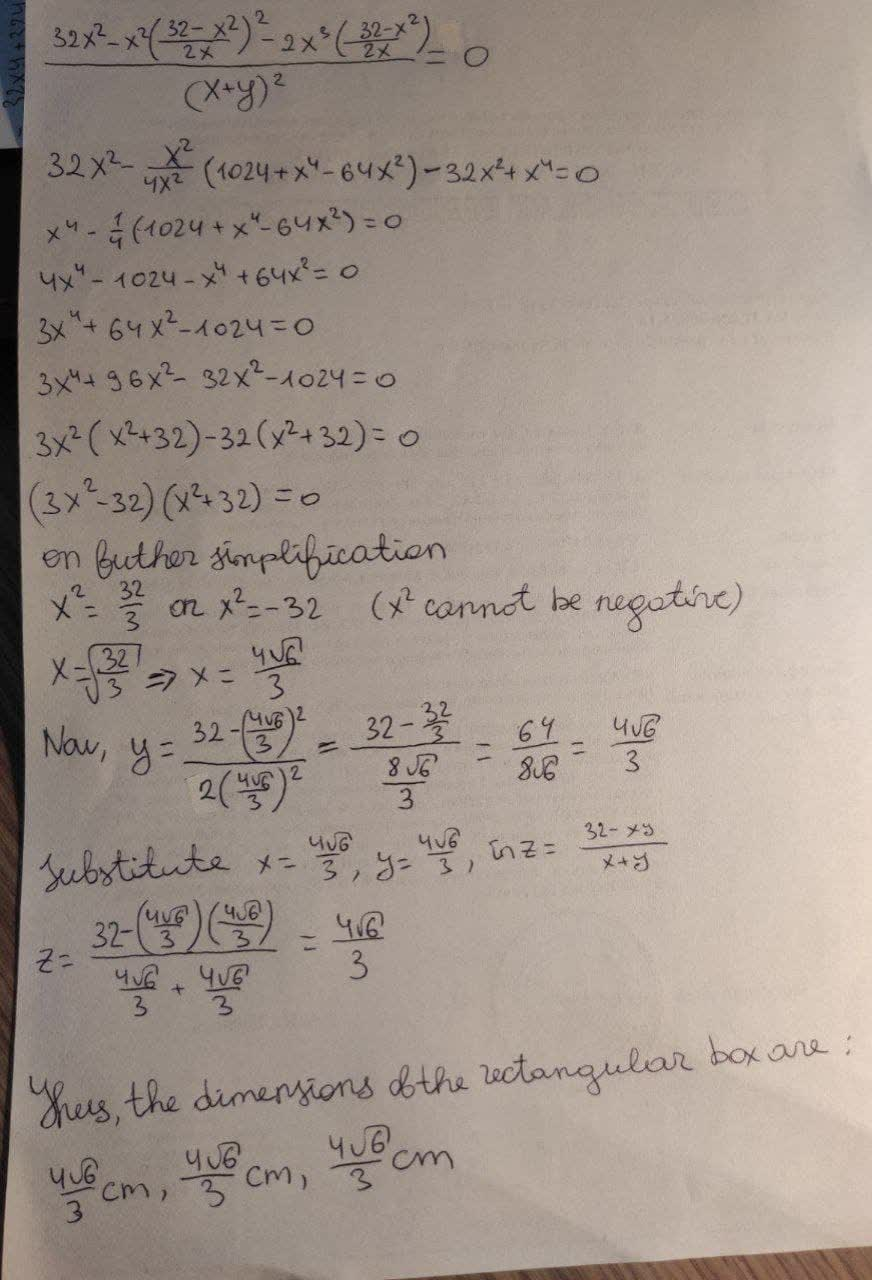# Multivariable optimization. Find the demisions of the rectangular box with largest volume if the total surface are is given as 64 cm^2Question
Multivariable functionsMultivariable optimization. Find the demisions of the rectangular box with largest volume if the total surface are is given as 64 $$\displaystyle{c}{m}^{{2}}$$2021-02-23### Relevant QuestionsMultivariable optimization question. Find three positive real numbers whose sum is one and the sum of their squares is a minimum.A surface is represented by the following multivariable function,
$$\displaystyle{f{{\left({x},{y}\right)}}}=\frac{{1}}{{3}}{x}^{{3}}+{y}^{{2}}-{2}{x}{y}-{6}{x}-{3}{y}+{4}$$
a) Calculate $$\displaystyle{f}_{{\times}},{f}_{{{y}{x}}},{f}_{{{x}{y}}}{\quad\text{and}\quad}{f}_{{{y}{y}}}$$
b) Calculate coordinates of stationary points.
c) Classify all stationary points.A surface is represented by the following multivariable function,
$$\displaystyle{f{{\left({x},{y}\right)}}}={x}^{{3}}+{y}^{{3}}-{3}{x}-{3}{y}+{1}$$
Calculate coordinates of stationary points.Comsider this, multivariable fucntion
$$\displaystyle{f{{\left({x},{y}\right)}}}={3}{x}{y}-{x}^{{2}}+{5}{y}^{{2}}-{25}$$
a)What is the value of f(-1,3)?
b)Find all x-values such that f(x,x)=0Consider this multivariable function. f(x,y)=xy+2x+y−36
a) What is the value of f(2,−3)?
b) Find all x-values such that f (x,x) = 0Consider this multivariable function. $$\displaystyle{f{{\left({x},{y}\right)}}}={y}{e}^{{{3}{x}}}+{y}^{{2}}$$
a) Find $$\displaystyle{{f}_{{y}}{\left({x},{y}\right)}}$$
b) What is value of $$\displaystyle{{f}_{{\times}}{\left({0},{3}\right)}}$$?A concert promoter produces two kinds of souvenir shirt, one kind sells for $18 ad the other for$25. The company determines, the total cost, in thousands of dollars, of producting x thousand of the $18 shirt and y thousand of the$25 shirt is given by
$$\displaystyle{C}{\left({x},{y}\right)}={4}{x}^{{2}}-{6}{x}{y}+{3}{y}^{{2}}+{20}{x}+{19}{y}-{12}.$$
How many of each type of shirt must be produced and sold in order to maximize profit?Let $$\displaystyle{z}{\left({x},{y}\right)}={e}^{{{3}{x}{y}}},{x}{\left({p},{q}\right)}=\frac{{p}}{{q}}{\quad\text{and}\quad}{y}{\left({p},{q}\right)}=\frac{{q}}{{p}}$$ are functions. Use multivariable chain rule of partial derivatives to find
(i) $$\displaystyle\frac{{\partial{z}}}{{\partial{p}}}$$
(ii) $$\displaystyle\frac{{\partial{z}}}{{\partial{q}}}$$.Average value over a multivariable function using triple integrals. Find the average value of $$\displaystyle{F}{\left({x},{y},{z}\right)}={x}^{{2}}+{y}^{{2}}+{z}^{{2}}$$ over the cube in the first octant bounded bt the coordinate planes and the planes x=5, y=5, and z=5Let $$\displaystyle{z}={e}^{{{x}^{{2}}+{3}{y}}}+{x}^{{3}}{y}^{{2}}$$, where $$\displaystyle{x}={t}{\cos{{r}}}{\quad\text{and}\quad}{y}={r}{t}^{{4}}$$. Use the chain rule fot multivariable functions (Cals III Chain Rule) to find $$\displaystyle\frac{{\partial{z}}}{{\partial{r}}}$$ and $$\displaystyle\frac{{\partial{z}}}{\partial}{t}{)}$$. Give your answers in terms of r and t only. Be sure to show all of your work.